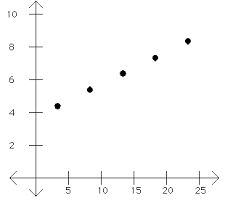# Kolmogorov Complexity & Minimum Description Length

Kolmogorov Complexity and the minimum description length (MDL) principle helps you to choose among alternate models. Model selection is a huge challenge in statistics, and MDL gives a generic solution by compressing data. The basic idea is that the optimal model is the most compressed model. Kolmogorov Complexity helps you choose a model by encouraging a simple hypothesis to explain the data.

Closely related to Occam’s razor, MDL states that the best explanation for a set of data is one that allows you to compress the data as much as possible; to encode it in the shortest way. The more a data set can be compressed, the more regularities appear, and the more can be learned from the data.

## Kolmogorov Complexity

“…given some data, the simplest hypothesis explaining it is preferable.” ~Rissanen (1989)

With MDL, Kolmogorov complexity is used to find the “simplest” hypothesis. Kolmogorov complexity is the shortest code which would cause a Turing machine to print out a set of numbers. For example, the following scatter plot shows a series of coordinates:These points could be described in several ways involving linear equations and lists of coordinate points on a Cartesian plane. However, the shortest way to describe the points is:

1. The coordinate of the first point,
2. The direction of the line,
3. Distance between points,
4. Total number of points.

The above “code” is therefore the Kolmogorov complexity for the above series of points.

In an ideal world, we could use the minimum description length principle to choose a model by using Kolmogorov Complexity. Comparing these shortest codes for different models, it would be easy to see which was the absolute shortest; in ideal MDL this model would be chosen.

This works theoretically because it doesn’t matter what (general purpose) computer language is used: the lengths of the shortest programs printed out will only differ by a constant, so the differences between programs for various models are stable.

There is just one problem with this method: Kolmogorov Complexity, while it would be incredibly useful if we could get a calculator to spit it out, is not computable. There isn’t any general-use computer program, or, theoretically, any possibility of writing one, that would find the shortest program that prints A for any data set or model A.

## Practical Minimum Description Length Theory

Since we can’t do what we’d like to do—summarize the results of every model in ‘shortest possible code’ and see which is shorter—we need to work with what we can. Rather than using general-purpose computer languages, we use more specific methods of summarizing our models; We use specific languages that, while they couldn’t describe anything, at least describe our models. We can use probability distributions to describe our data, and linear regression models to determine what fits best.

## References

Singh, Aarti. 10-704 Information Processing and Learning Class Notes: Lecture 13: Minimum Description Length. Retrieved from http://www.cs.cmu.edu/~aarti/Class/10704/lec13-MDL.pdf on April 26, 2018
Hansen, Mark H., Yu, Bin. Model Selection and the Principle of Minimum Description Length.